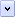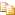Methods are implemented as functions or subroutines in your Windows® Script Component file.

# Procedures

### To expose a method

1. Create a <public> element as a child of the <component> element.

2. In the <public> element, include a <method> element. The method element can optionally include one or more <parameter> elements to define the method's parameters.

3. Write a procedure in any scripting language to implement the function. Place the procedure in a <script> element outside the <implements> element but within the <component> element. Be sure the function name matches the functionName, or if you did not specify a functionName, the methodName name you specified in the <method> element.

For example, the following example shows a fragment from a script component file with two methods, factorial and getRandomNumber.Note

A CDATA section is required to make the script in the <script> element opaque. For details, see Script Component Files and XML Conformance.Copy Code
```<public>
<method name="factorial"/>
<method name="random" internalName="getRandomNumber">
<parameter name="upperBound"/>
<parameter name="seed"/>
</method>
</public>

<script language="VBScript">
Function factorial(n)
<![CDATA[
If isNumeric(n) Then
If n <= 1 Then
factorial = 1
Else
factorial = n*factorial(n-1)
End If
Else
factorial = -2   ' Error code.
End If
End Function

Function getRandomNumber(upperBound, seed)
getRandomNumber = Cint(upperBound * Rnd(seed) + 1)
End Function
]]>
</script>```

You can specify a default method for a script component so the host application can invoke the method without explicitly calling it. For example, if you have exposed a method called factorial and marked it as the default, you can call it in the following ways in Visual Basic:Copy Code
```Set component = CreateObject("component.MyComponent")
n = component.factorial(4)   ' Calls factorial method explicitly.
n = component(4)   ' Calls factorial method as default.```

To specify a default method, include an attribute assigning a special dispatch identifier (a dispid) to the method. For more information about dispids, see Exposing Events.

### To specify a default method

• In the <method> element, include the attribute dispid="0", as shown in the following example:Copy Code
```<public>
<method name="factorial" dispid="0"/>
</public>```Note

This technique can be used to assign either a default method or a default property, but not both. There can be only one element in the script component with the dispid of zero.

Exposing Properties

#### Concepts

Exposing Events
Script Component File Contents

Home